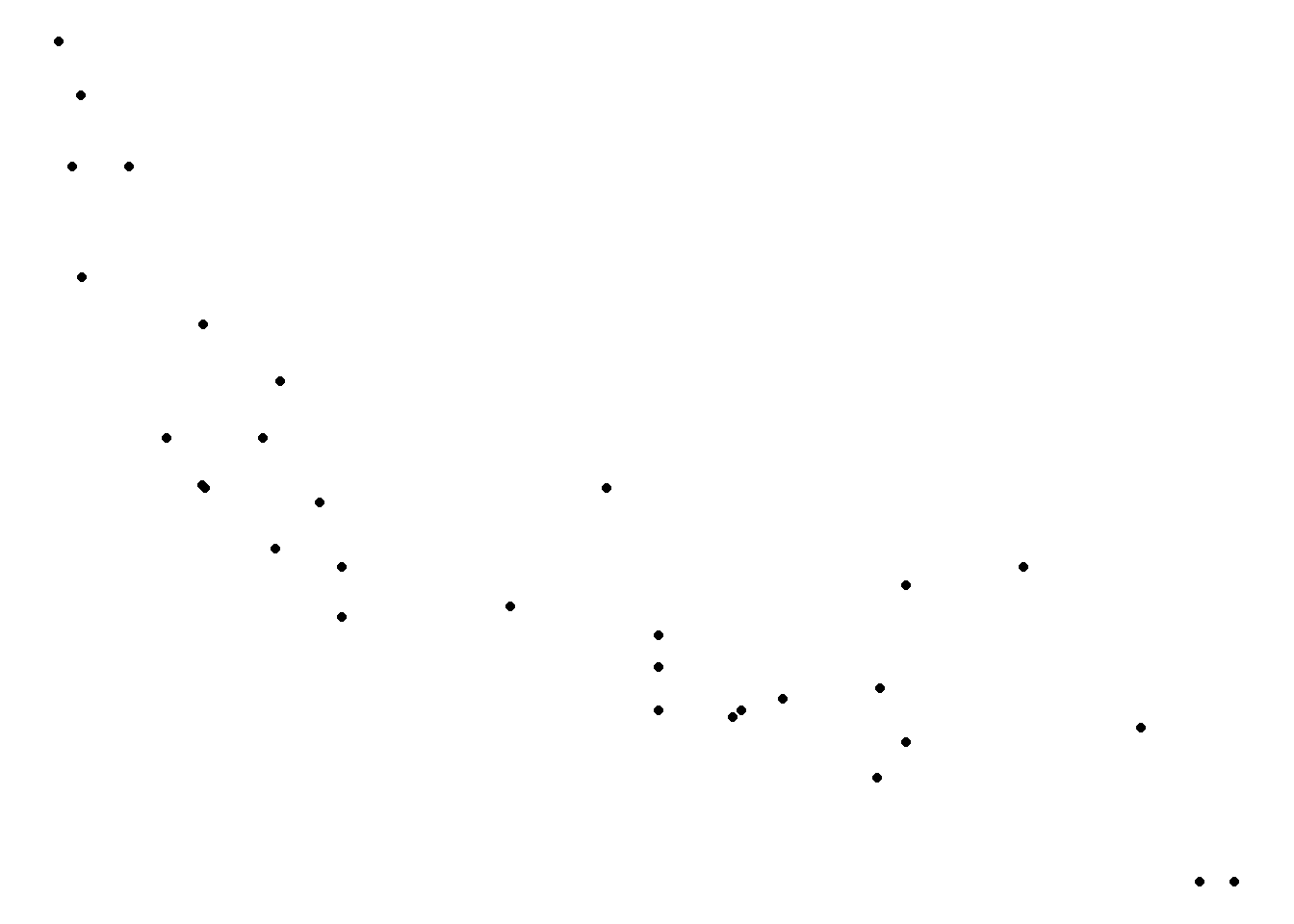# Chapter 14 Themes

## 14.1 Introduction

In this final chapter, we will learn to modify the appearance of all non data components of the plot such as:

• axis
• legend
• panel
• plot area
• background
• margin
• facets

## 14.2 Basic Plot

We will continue with the scatter plot examining the relationship between displacement and miles per gallon from the the mtcars data set.

``````p <- ggplot(mtcars) +
geom_point(aes(disp, mpg))
p``````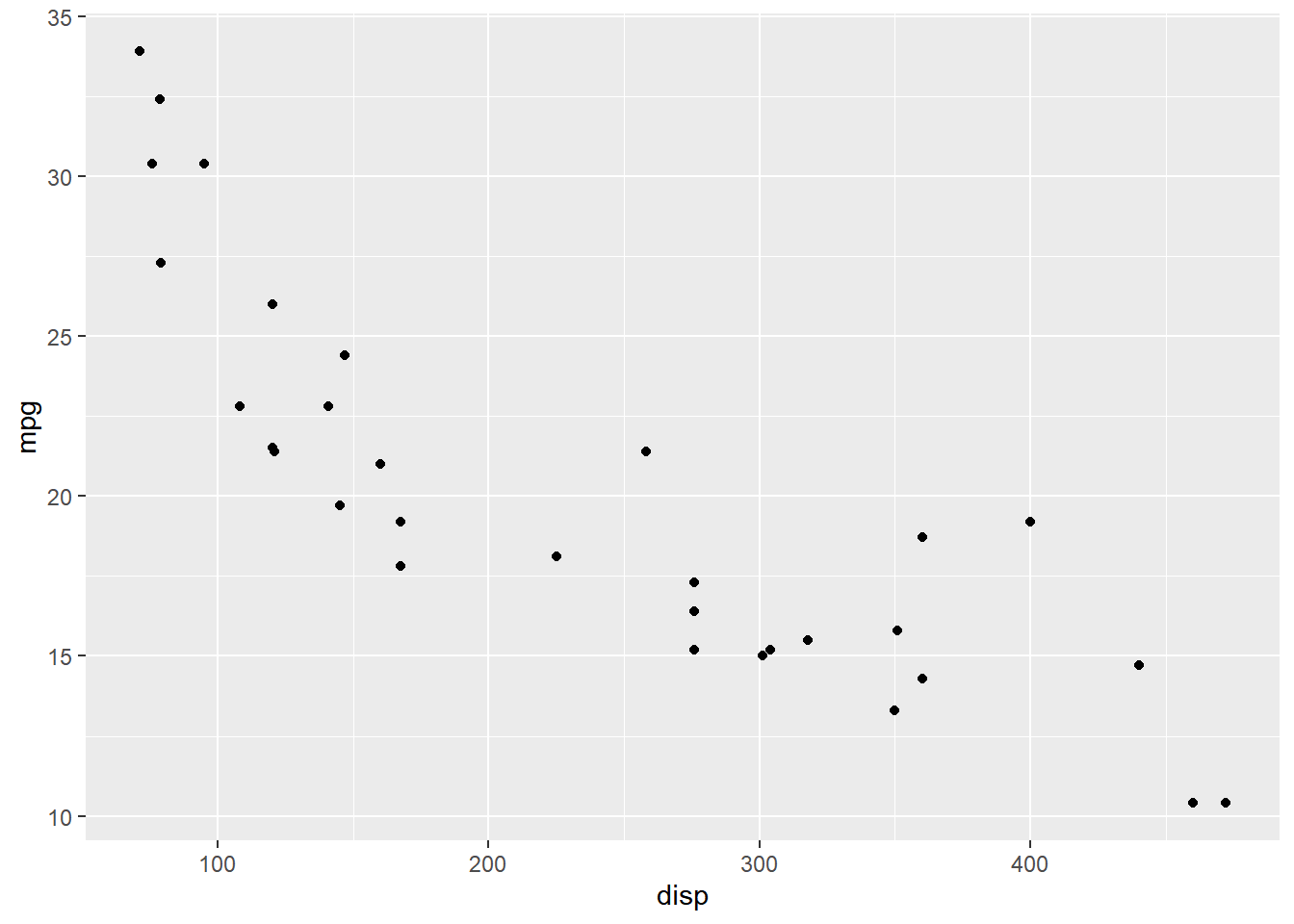## 14.3 Axis

### 14.3.1 Text

The `axis.title.x` argument can be used to modify the appearance of the `X` axis. In the below example, we modify the color and size of the title using the `element_text()` function. Remember, whenever you are trying to modify the appearance of a theme element which is a text, you must use `element_text()`.

You can use `axis.title.y` to modify the `Y` axis title and to modify the title of both the axis together, use `axis.title`.

``p + theme(axis.title.x = element_text(color = "red", size = 10, face = "italic"))``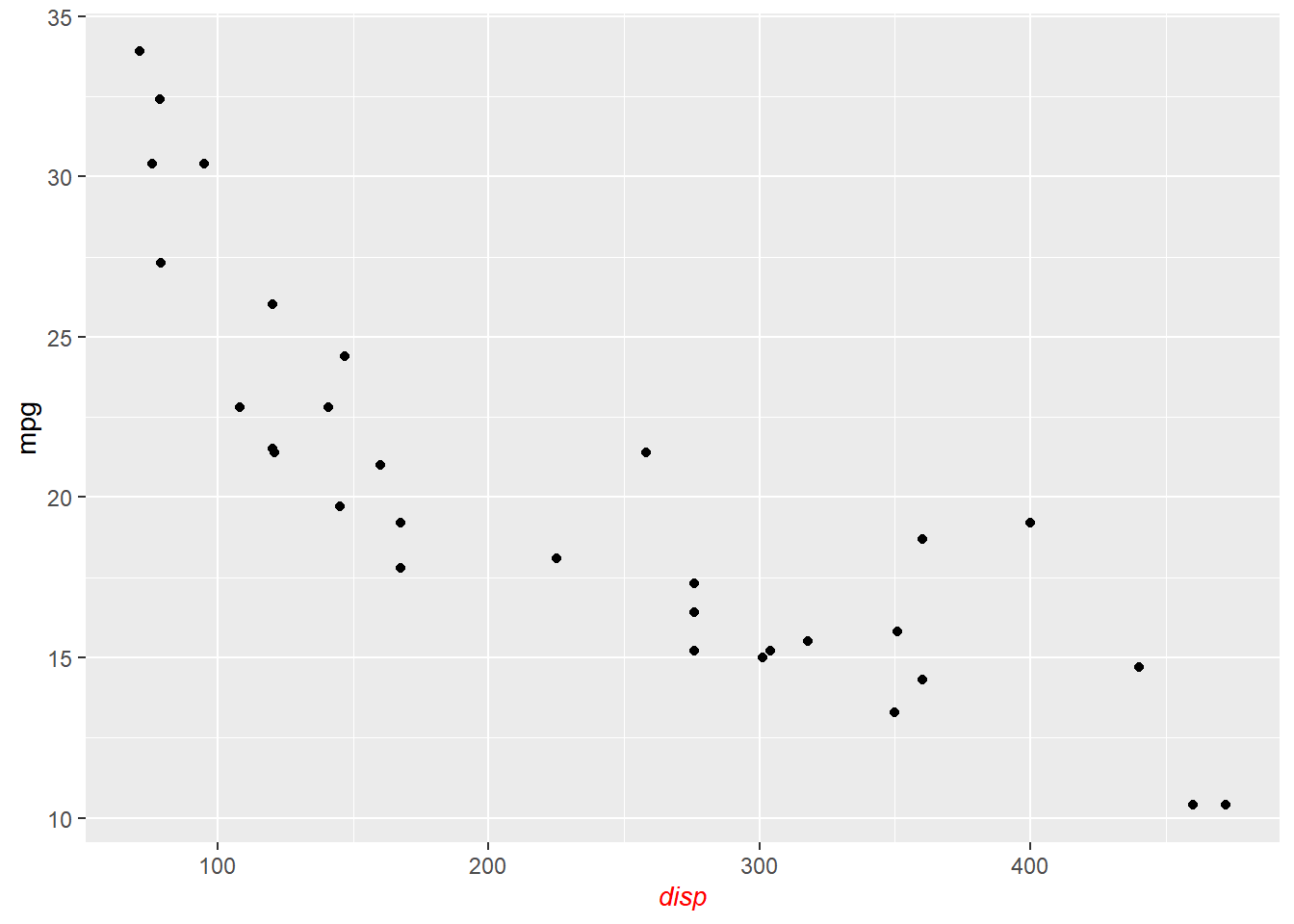### 14.3.2 Ticks

To modify the appearance of the axis ticks, use the `axis.ticks` argument. You can change the color, size, linetype and length of the ticks using the `element_line()` function as shown below.

``````p + theme(axis.ticks = element_line(color = 'blue', size = 1.25, linetype = 2),
axis.ticks.length = unit(1, "cm"))``````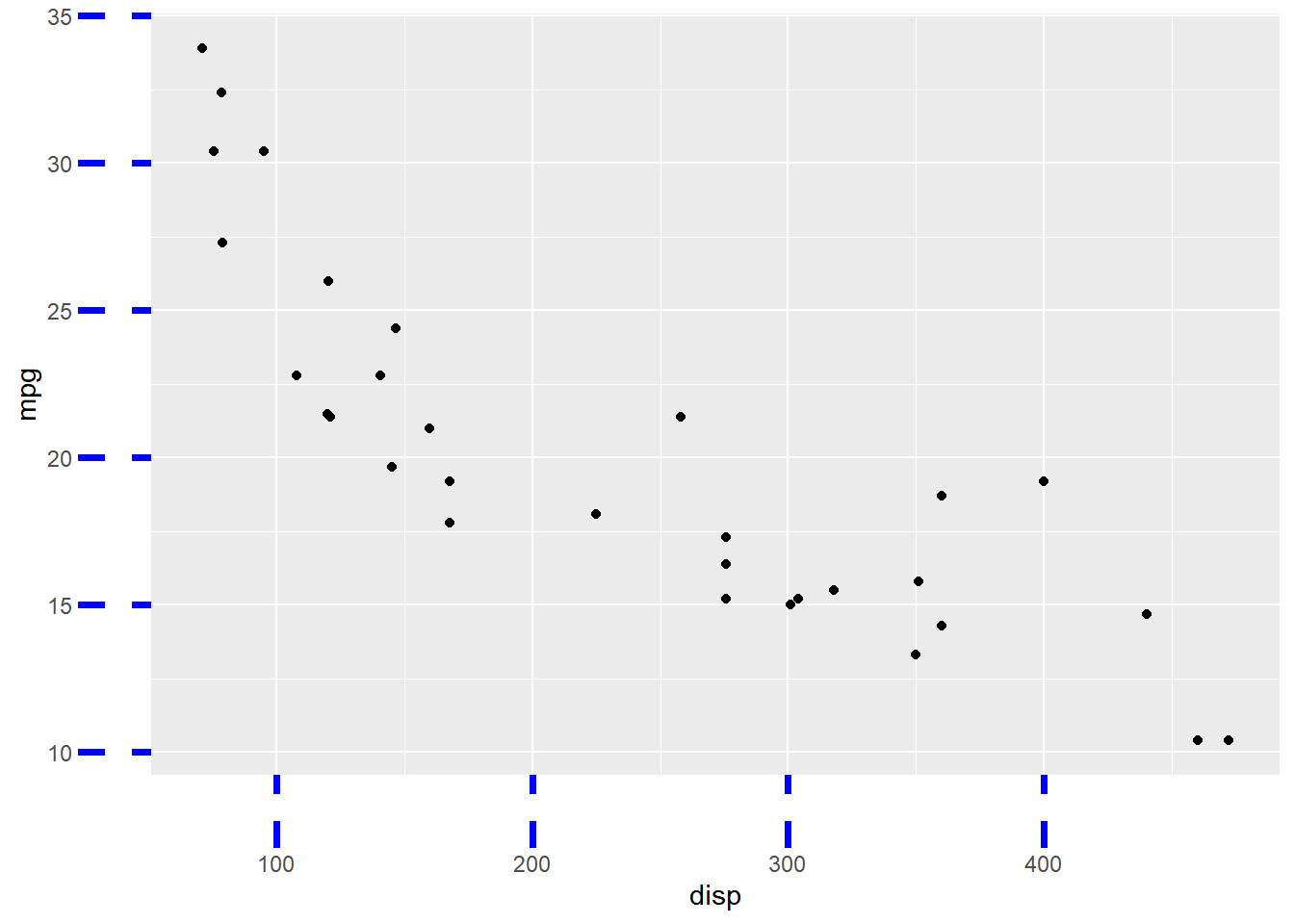### 14.3.3 Line

The `axis.line` argument should be used to modify the appearance of the axis lines. You can change the color, size and linetype of the line using the `element_line()` function.

``p + theme(axis.line = element_line(color = 'red', size = 1.5, linetype = 3))``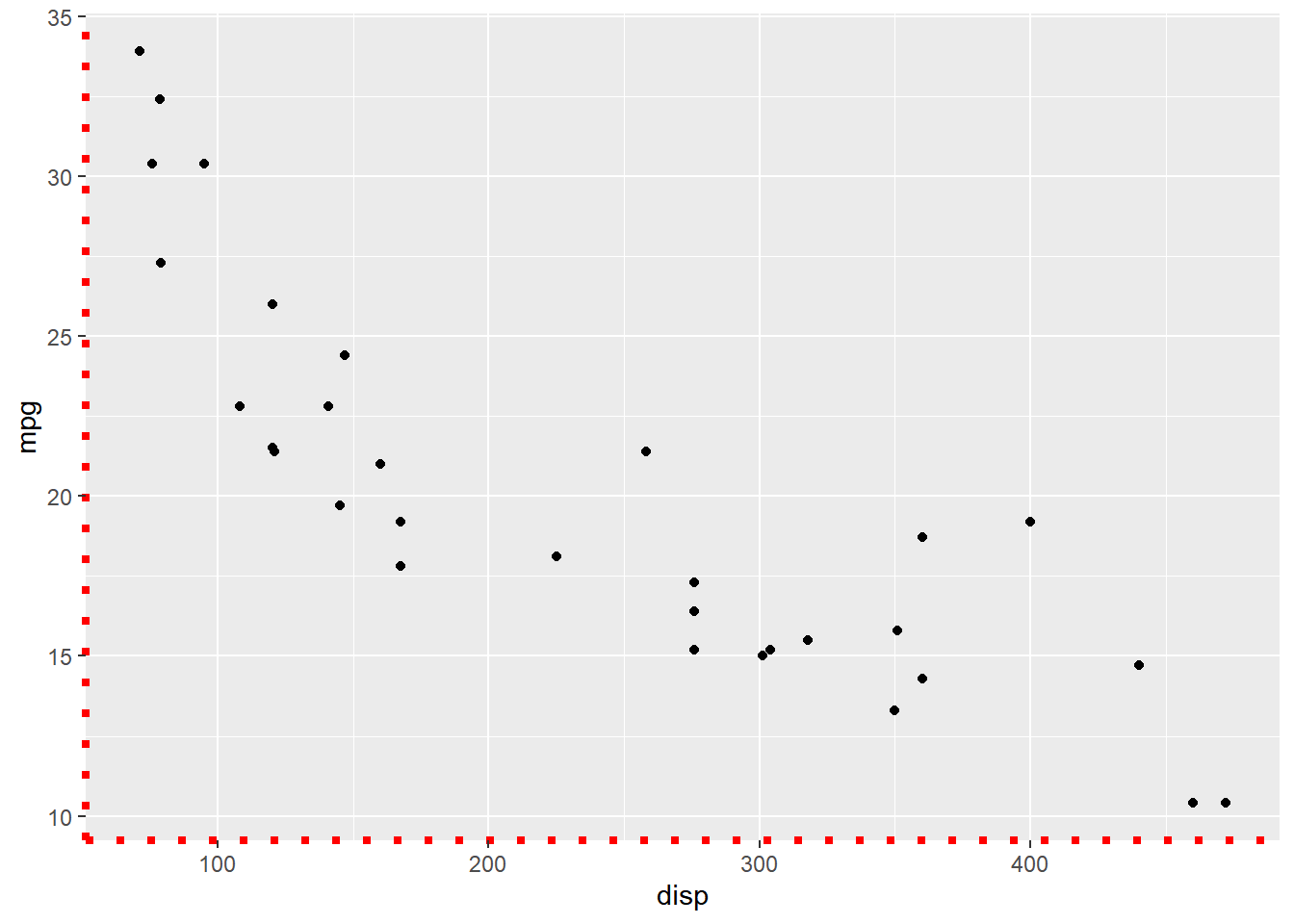## 14.4 Legend

Now, let us look at modifying the non-data components of a legend.

``````p <- ggplot(mtcars) +
geom_point(aes(disp, mpg, color = factor(cyl), shape = factor(gear)))
p``````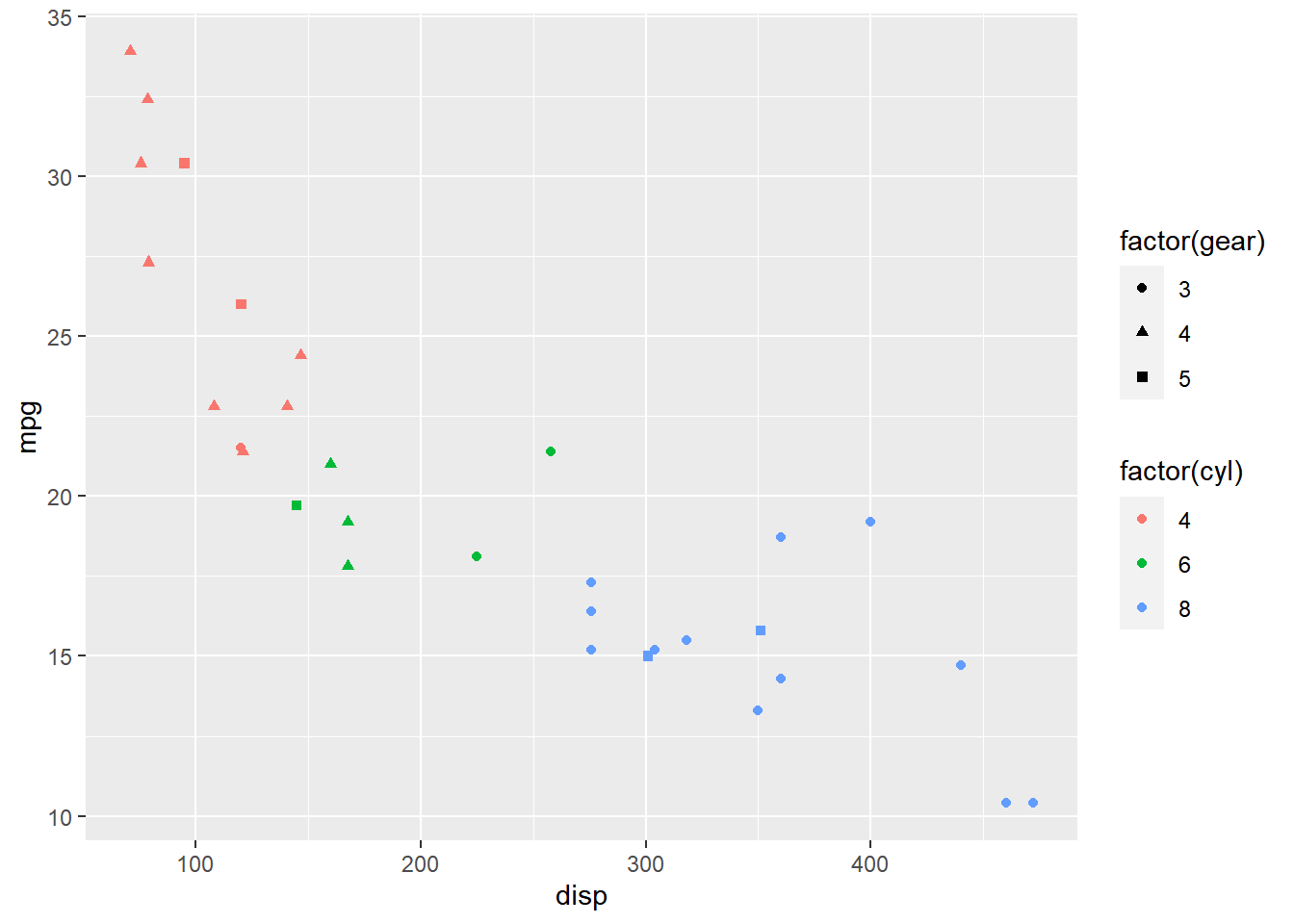### 14.4.1 Background

The background of the legend can be modified using the `legend.background` argument. You can change the background color, the border color and line type using `element_rect()`.

``````p + theme(legend.background = element_rect(fill = 'gray', linetype = 3,
color = "black"))``````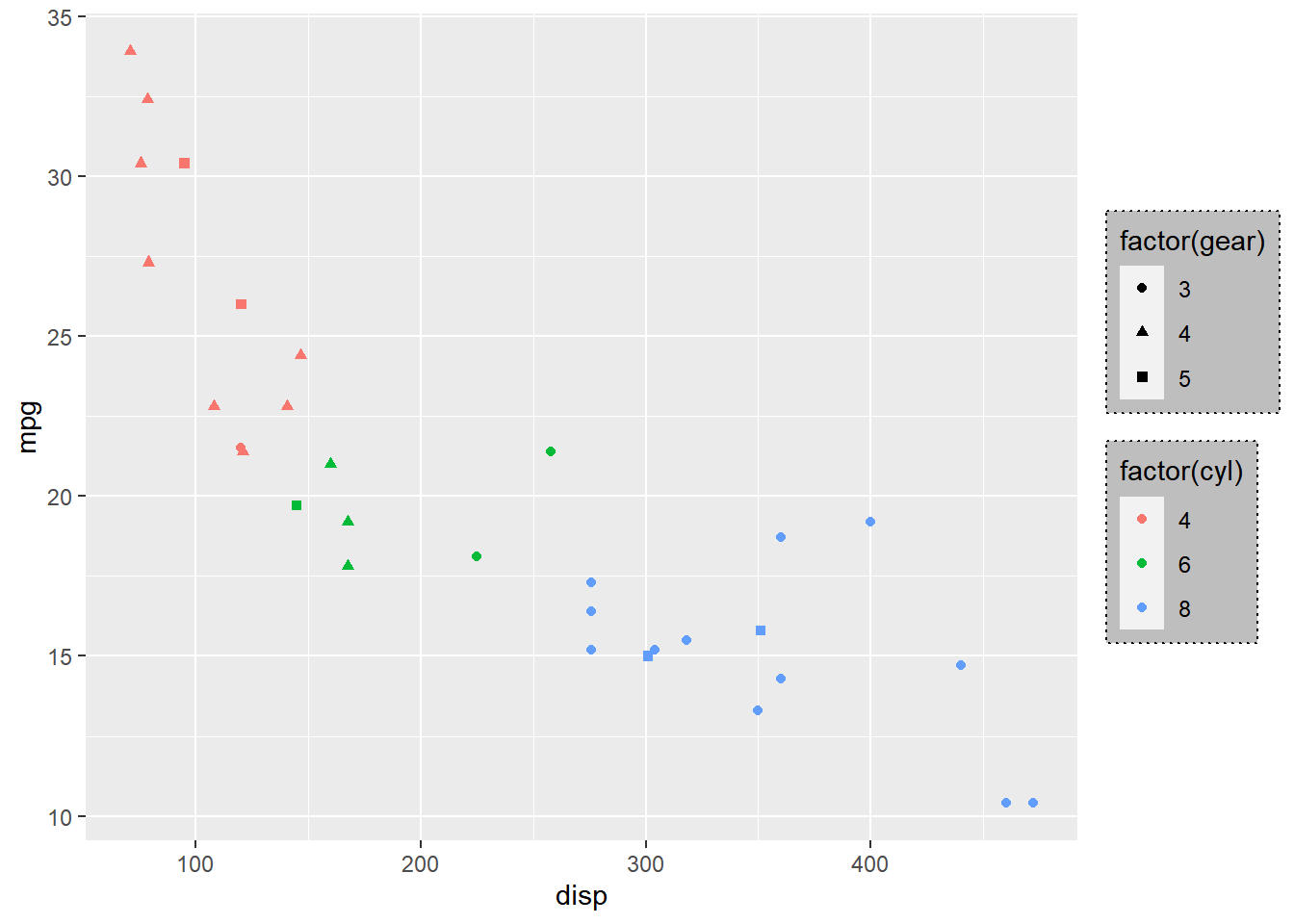### 14.4.2 Text

The appearance of the text can be modified using the `legend.text` argument. You can change the color, size and font using the `element_text()` function.

``p + theme(legend.text = element_text(color = 'green', face = 'italic'))``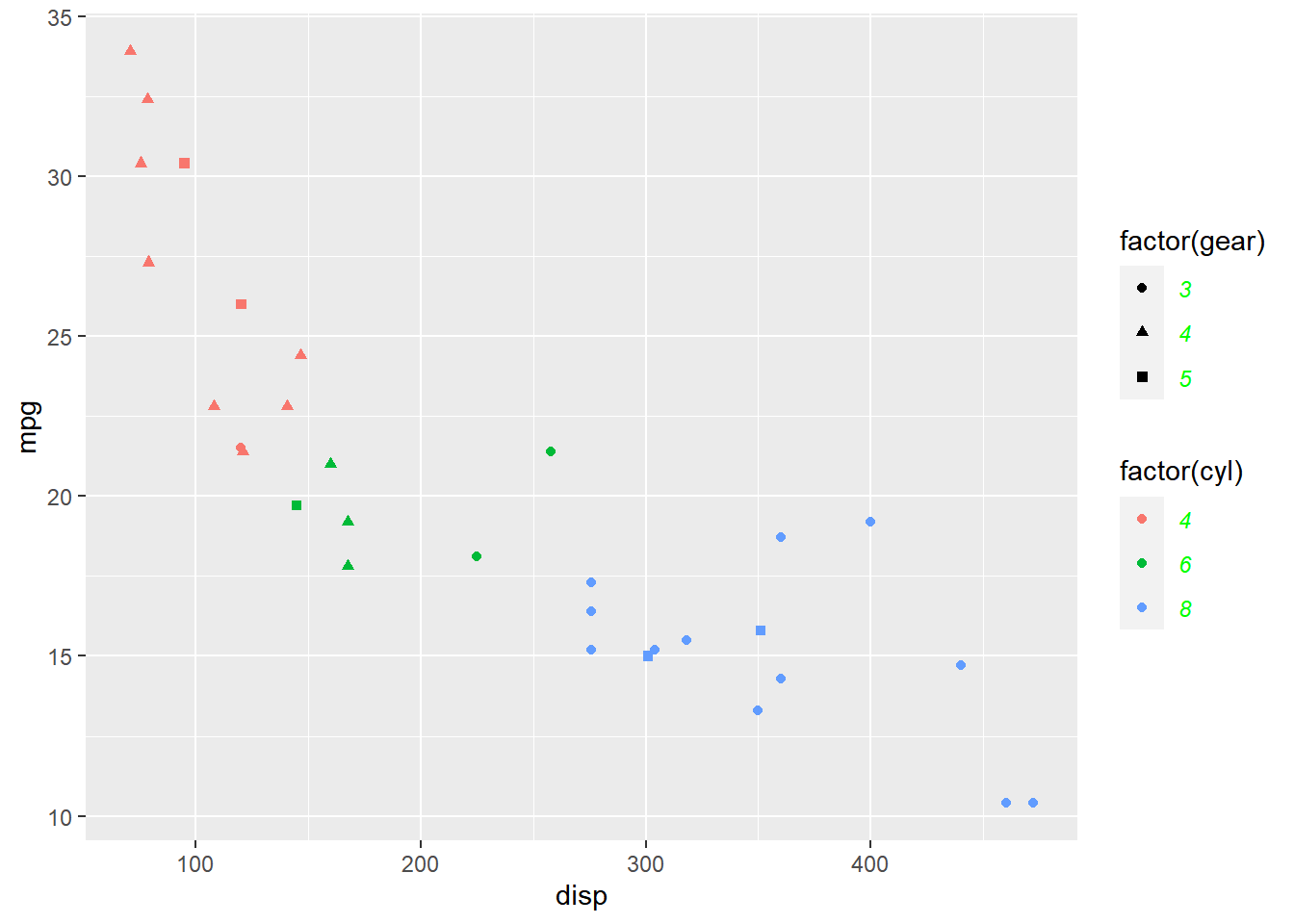### 14.4.3 Title

The appearance fo the title of the legend can be modified using the `legend.title` argument. You can change the color, size, font and alignment using `element_text()`.

``````p + theme(legend.title = element_text(color = 'blue', face = 'bold'),
legend.title.align = 0.1)``````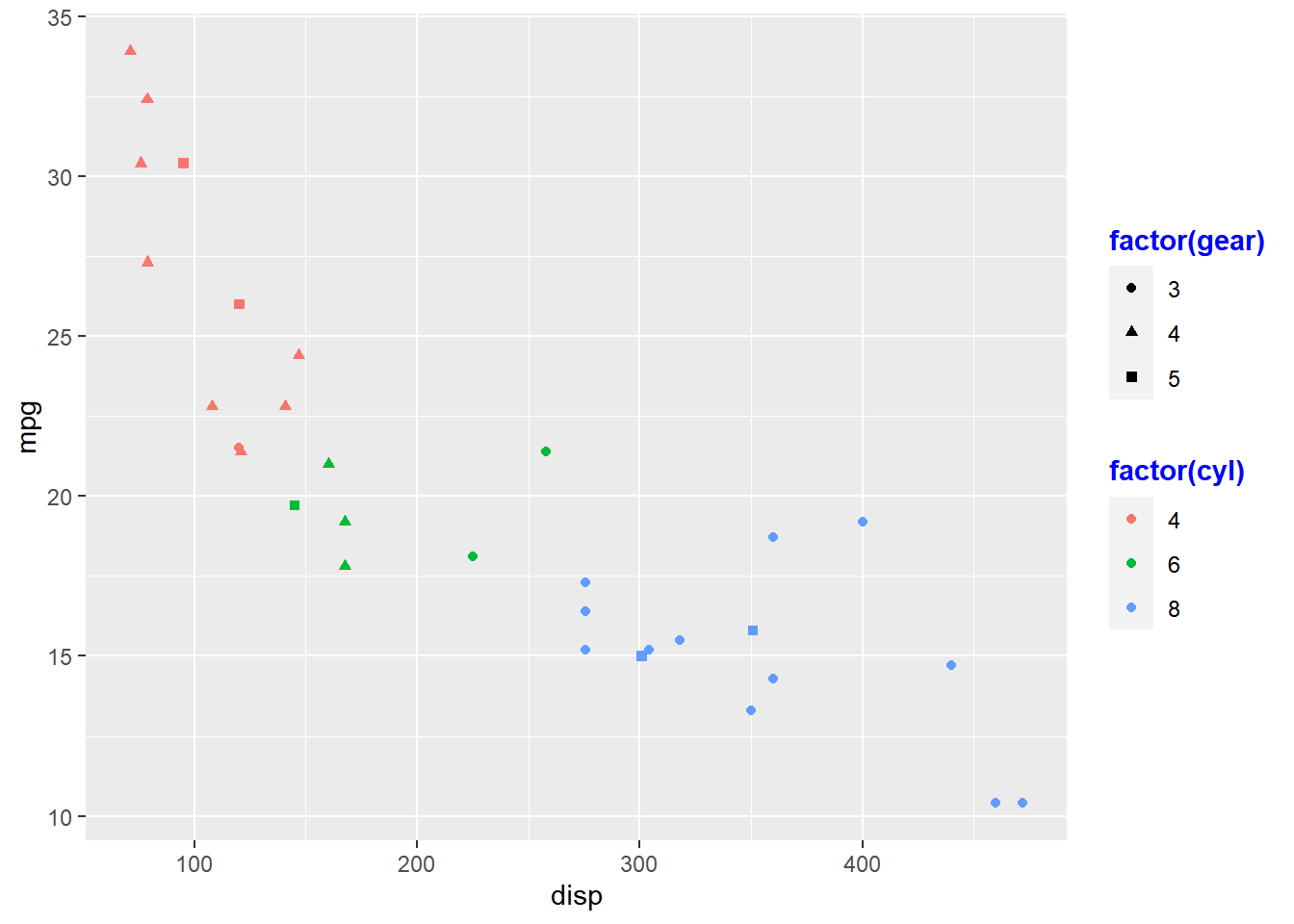### 14.4.4 Position

The position and direction of the legend can be changed using:

• `legend.position`
• and `legend.direction`
``p + theme(legend.position = "top", legend.direction = "horizontal")``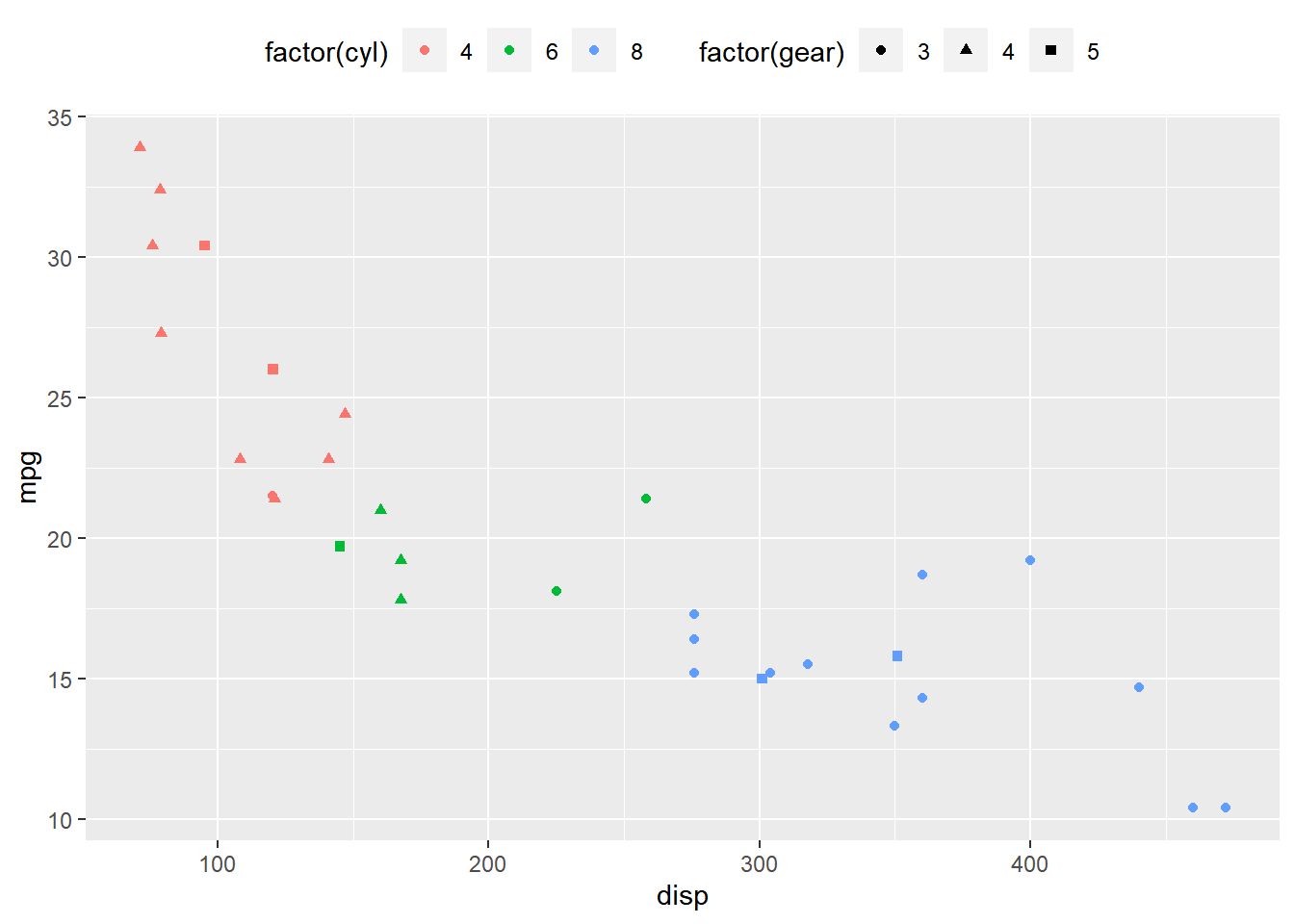## 14.5 Themes

### 14.5.1 Classic Dark on Light

``````ggplot(mtcars) +
geom_point(aes(disp, mpg)) +
theme_bw()``````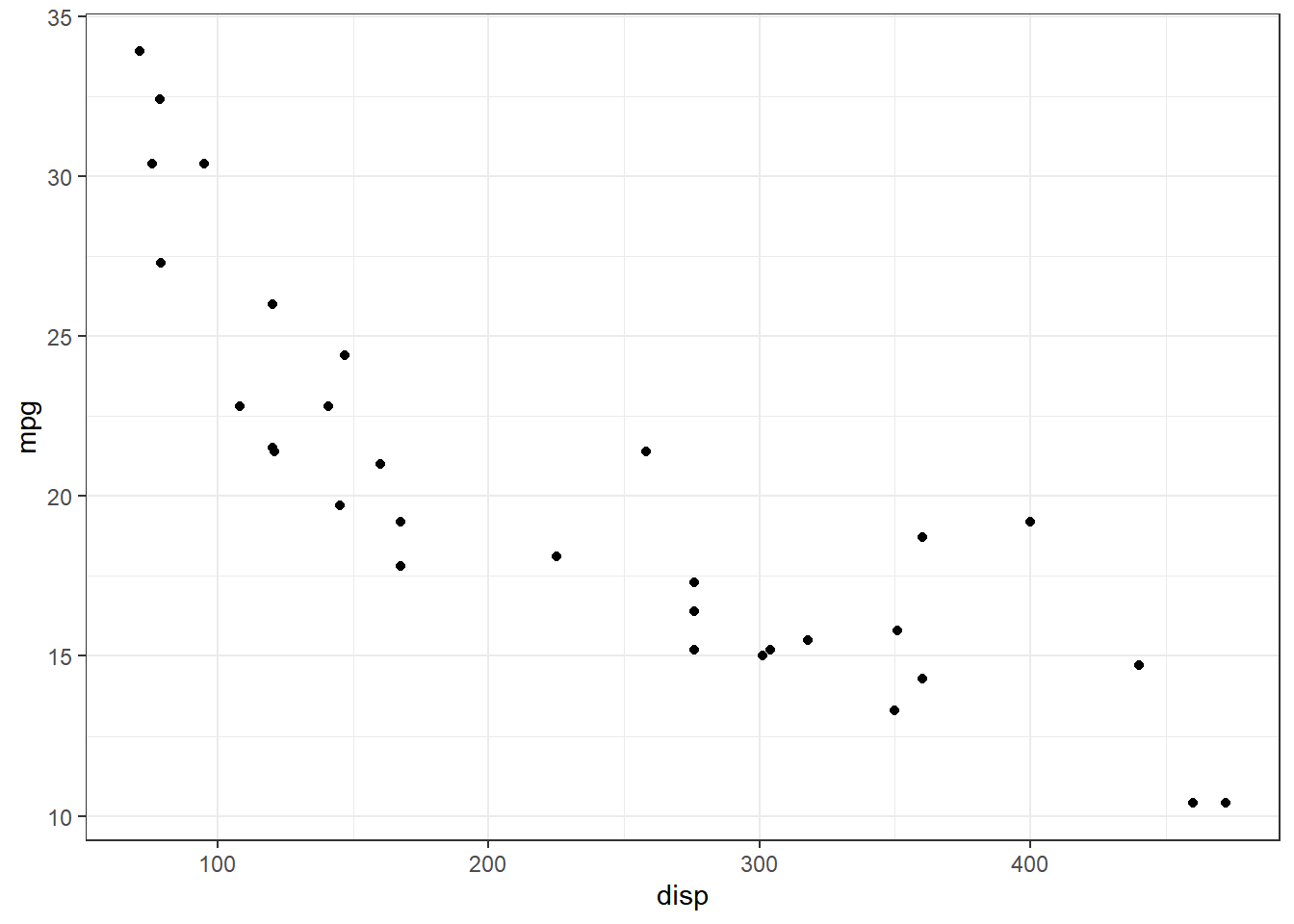### 14.5.2 Default Gray

``````ggplot(mtcars) +
geom_point(aes(disp, mpg)) +
theme_gray()``````### 14.5.3 Light

``````ggplot(mtcars) +
geom_point(aes(disp, mpg)) +
theme_light()``````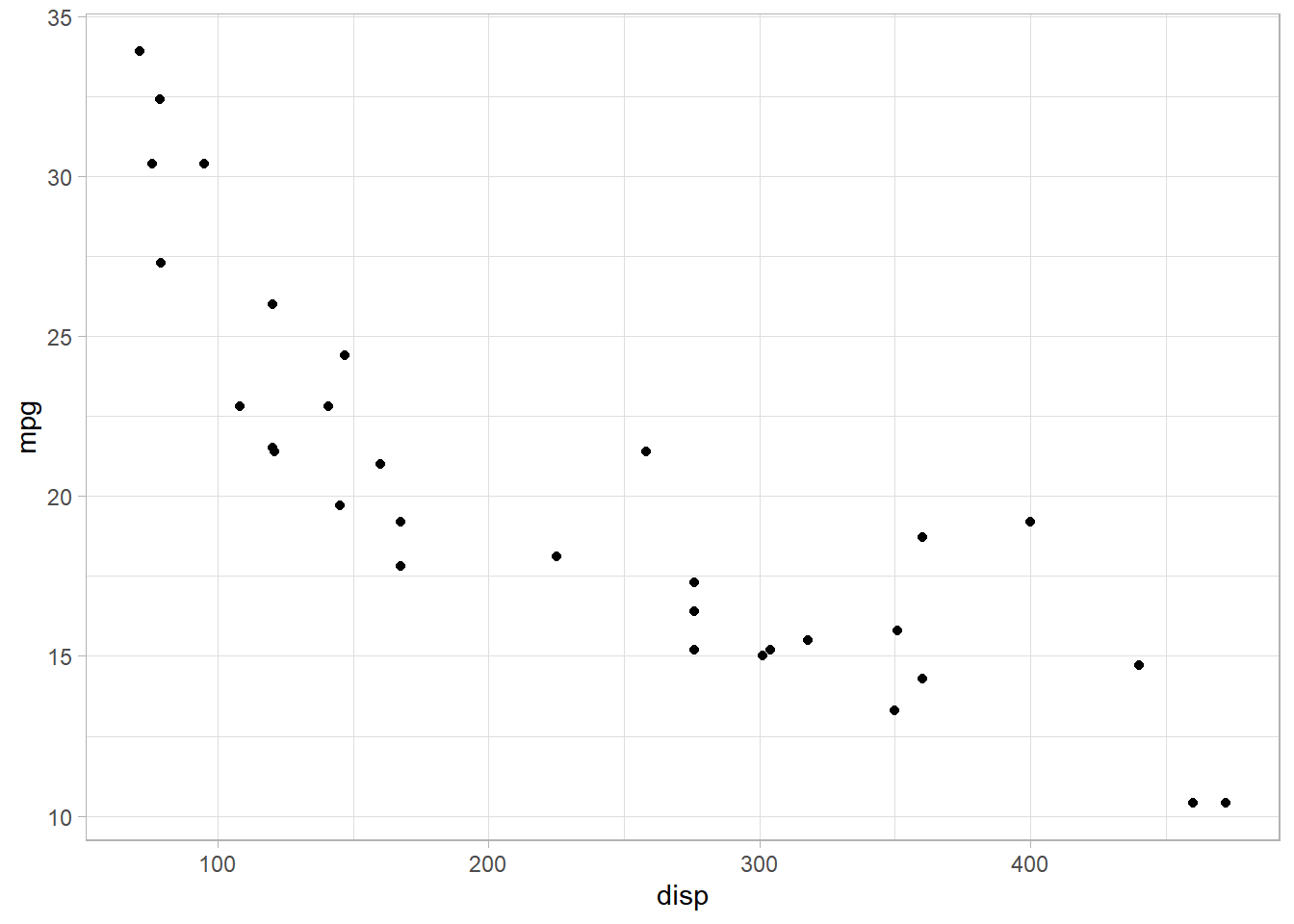### 14.5.4 Minimal

``````ggplot(mtcars) +
geom_point(aes(disp, mpg)) +
theme_minimal()``````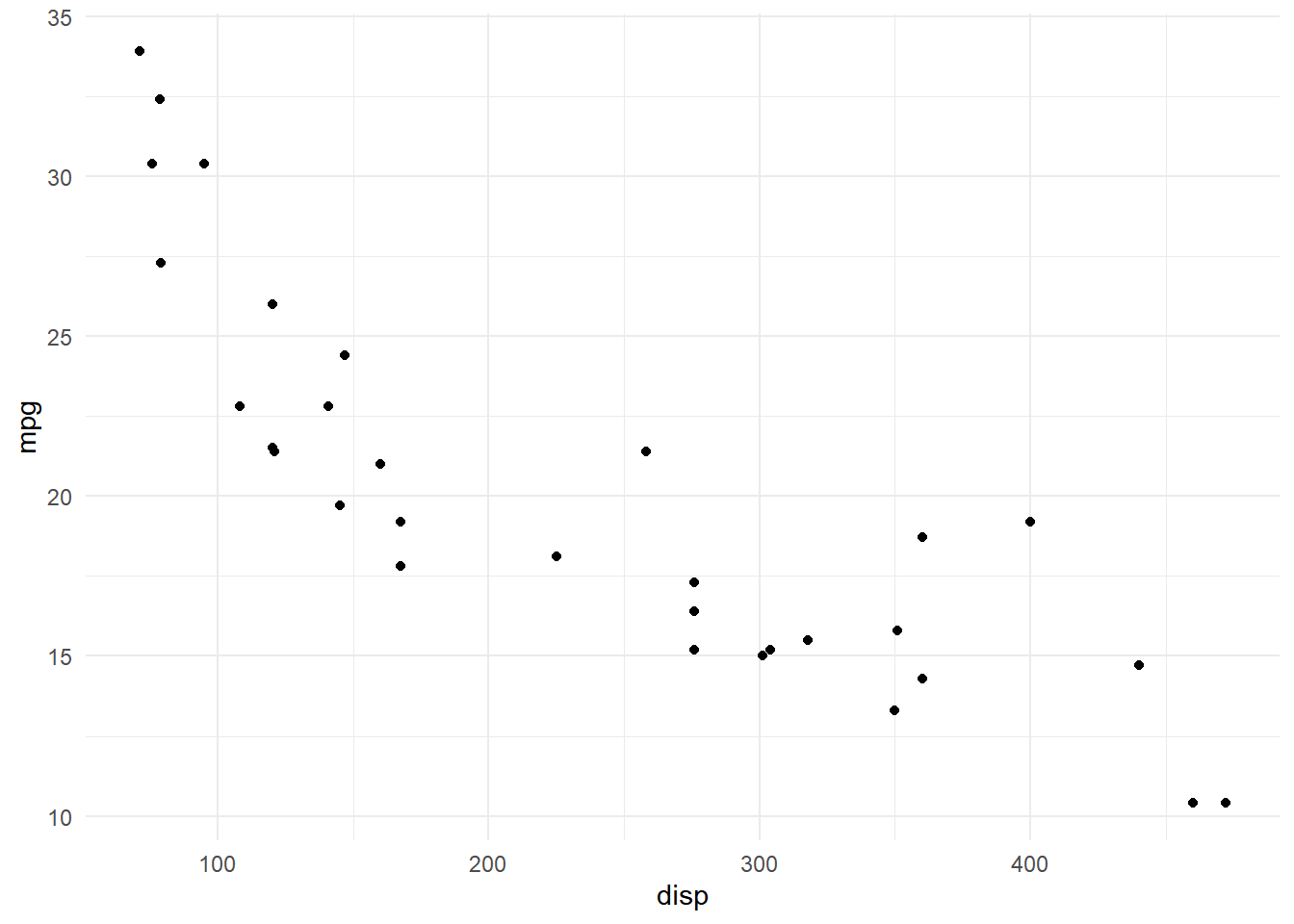### 14.5.5 Dark

``````ggplot(mtcars) +
geom_point(aes(disp, mpg)) +
theme_dark()``````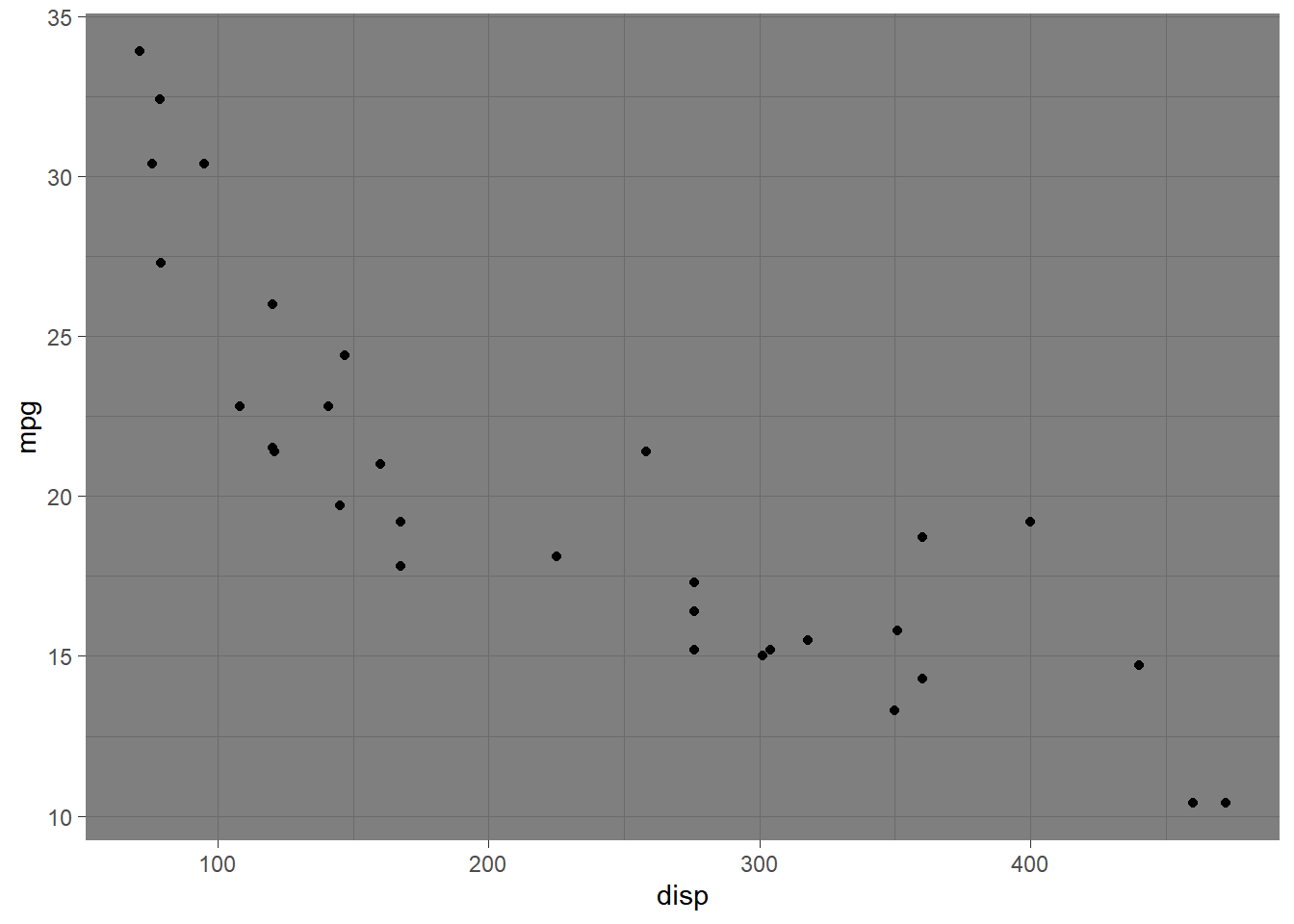### 14.5.6 Classic

``````ggplot(mtcars) +
geom_point(aes(disp, mpg)) +
theme_classic()``````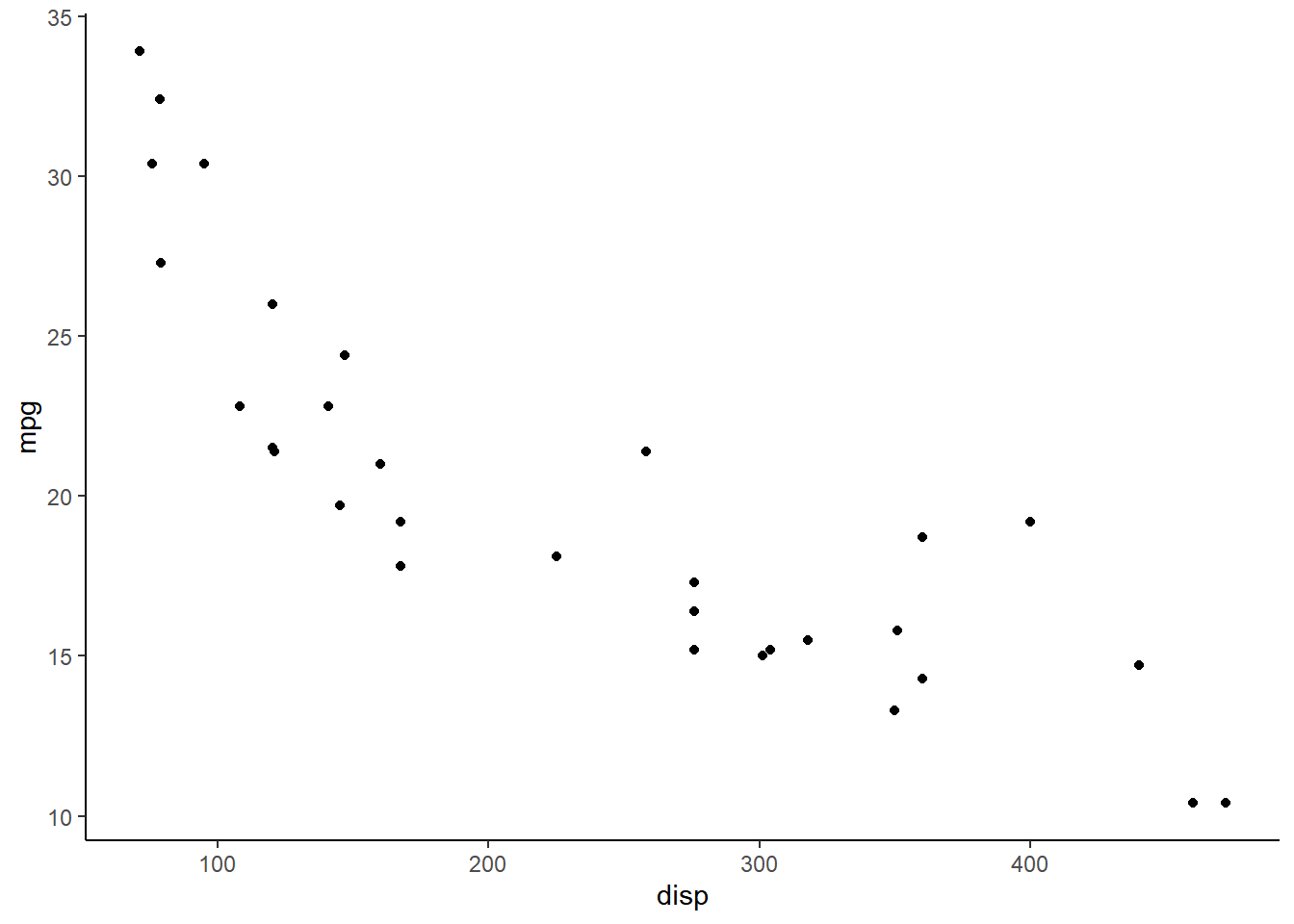### 14.5.7 Void (Empty)

``````ggplot(mtcars) +
geom_point(aes(disp, mpg)) +
theme_void()``````